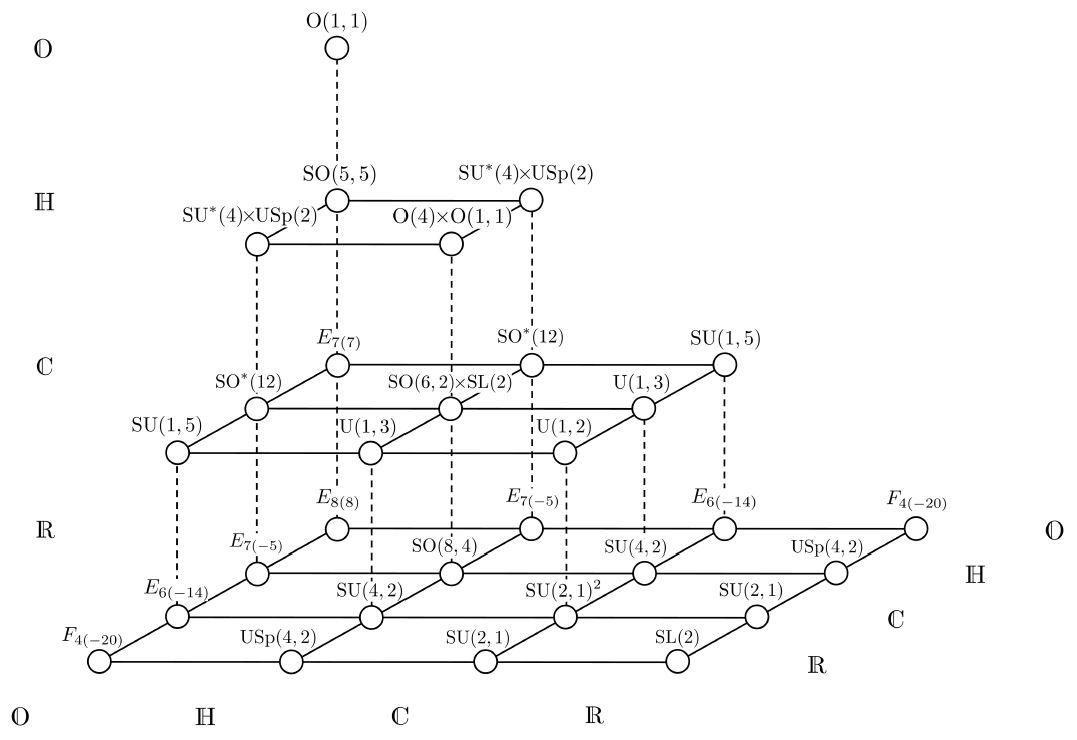# nLab magic pyramid

Contents

### Context

#### Gravity

gravity, supergravity

supersymmetry

# Contents

## Idea

The classification of pure supergravity theories in dimensions 3,4,6,10 and 4,5,7,11 is parameterized by the real normed division algebras, while the classification of super Yang-Mills theories is parameterized by pairs of real normed division algebras, see at supersymmetry and division algebra and at spin representation.

Combining this yields a classification of the extended super Einstein-Yang-Mills theories by triples of real normed division algebras. called a magic pyramid of supergravity theories (ABDHN 13).(from ABDHN 13, p. 3)

In this diagram vertical axis labels the spacetime division algebra (hence vertical label $\mathbb{R}$ corresponds to $d = 2+1$, vertical label $\mathbb{C}$ to $d = 3+1$, $\mathbb{H}$ to $d = 5+1$ and $\mathbb{O}$ to $d = 9+1$) , while the horizontal axes label the algebras associated with the number of left and right supersymmetries. The entries display the corresponding U-duality groups. Each horizontal level is a “magic square”.

based on